Views

# Introduction :

Ohms law  states that the current flowing through a conductor  is directly proportional to the potential difference across the ends of the conductor  . ohms law  is of wide importance in the field of electricity. The law helped physicists to not to understand the concept of electric current through a conductor but also helped to them to explain or evaluate different phenomenons taking place inside the  conductor during the flow of electric current.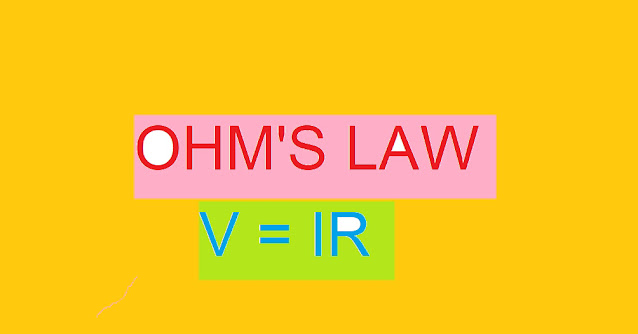ohms law

ohm also have a good hold in explaining the concept of resistance. Resistance as the name itself indicates that it resists something. Here we are dealing with electric current , so it means resisting the flow of current. In today's article I would like to share with you an interesting information related to ohms law  including the it's derivation from different concepts as well.

Without wasting my most of the time in introduction let me introduce you first , Hi there, my name is Tajamul fayaz and I'm gonna share with you an information related to ohms law  .

## ohms law :

This basic law was discovered by George Simon Ohm in 1828 . According to ohms law , that the current flowing through the conductor is proportional directly to the potential difference (v )  across the ends of the conductor. provided the physical conditions like temperature, pressure ,strain etc of the conductor remains unchanged.

That is ;

I ∝ V

V = RI

or       V/I  = R

What is R, V and I ? this question may be circulating in your mind. Don't worry, it is nothing but letters used to denote Resistance, Potential difference or voltage and electric current .

More important thing to know about R is that it the "constant of proportionality". The value of R depends upon the nature of the material including the dimensions and temperature. It does not depend on the values of V or I means neither on potential difference nor on the value of Electric current.

### Verification of  law :

ohms law can be verified by a simple procedure which ohm had used during its experiment. The method used to verify this law is known as "Voltmeter - Ammeter method". ohm suggested that a battery should be connected across the ends of the conductor though a rheostat, ammeter and key. In the present figure conductor is denoted as XY. Voltmeter across the conductor measures the potential difference across the conductor.

When key is closed, current flows through the conductor. Readings of voltmeter and ammeter are noted for different positions of rheostat. It is observed that V/I  = Constant. Thus ohms law is verified.

#### V-I characteristics :

V-I characteristics is not a tough concept for you to understand. This concept is basically based on the variation of potential difference (V)  and Electric current (I). Even you can perform this experiment by simply observing the variation in these two values of V and I.  This concept is represented in the form of a straight line having a constant slope = V/I = R.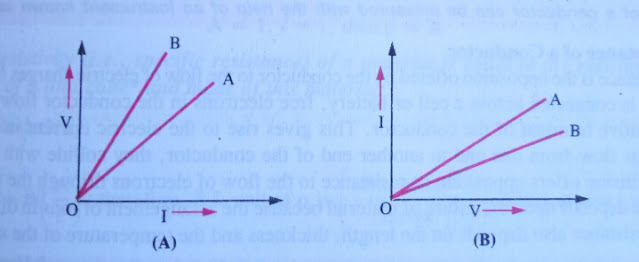V-I characteristics

It should be remembered that the slope of V-I characteristics curve means higher Resistance. So , we can say that the conductor B has higher resistance than the Resistance of conductor A. Similarly, V-I graph is shown in the figure B. Slope of V-I characteristics = I/V = 1/R . Thus , resistance of a conductor is inversely proportional to the slope of V-I curve .

Thus,  resistance of a conductor is large whose slope of V-I curve is small. Hence, resistance of conductor B is higher than the resistance of conductor A.

#### Derivation of  law from first principles :

ohms law - George Simon ohm  not only observed ,verified and published it but derived the law from other related concepts.  The first successful derivation of this law was from first principles.

how to derive? let's study the below given paragraph related to the derivation of ohms law

Let Vd be the drift velocity of electrons through a section of the conductor of length L and cross sectional area A. V is the potential difference across the section of the conductor, and E is electric field.

The Electric current in the conductor is given as;

I = neAVd

the symbol "n" denotes the number of electrons per unit volume in the conductor .

But magnitude of drift velocity,

Vd =  eEτ/m     = eVτ/ml

∴          I = neA (eVτ/ml)

= ne² (Aτ/ml) V.

or             V/I    =   ml/ne²Aτ = Constant  = R ( Resistance of the conductor)

This is ohms law

Where ,                    R  = ml/ne²Aτ = (m/ne²τ) l/A

#### Microscopic form of ohm law :-

The Microscopic form of ohms law  is an important concept to know.

As we know that the current is equal to

IneAVd      ......................(i)

But drift velocity,  Vd = eEτ/m

Therefore,         I  =  neA(eEτ/m)

= ne²AEτ/m

or                               I/A  =  ne²Eτ/m     ...............(ii)

Now               I/A   =  J  and  m/ne²τ = ρ

Where the letter "J" denotes the current density and "ρ" denotes the resistivity of the substance.

Therefore,  equation (ii)  becomes;

J    =     (1/ρ) E

But                1/ρ  =    σ

Where, the symbol  "σ"  is the conductivity of the substance.

Therefore,          J = σE

which is the microscopic form of ohms law

Again from equation (i),  we get;

Vd  =  I/Ane  = J/ne          ( ∵ I/A =J)

Using above equation, we get;

Vd    =     σE/ne

Numerical illustration:

Find drift velocity of electron in a conductor of conductivity  σ = 5.8 × 10 ̄⁷ W  ̄¹ m ̄¹, length 1m having potential difference of 200 v across its ends. Take electron density as   8.5  ×  10 ²***** m ̄³.

Solution:

using,       Vd    =   σE/ne

we get ,

Vd  = (5.8 × 10⁷) (200)/8.5 × 10²* × 1.6 × 10 ̄¹⁹

=  0.86 m s ̄¹ .

#### Limitations of the Law (ohm) :

As we know ohms law  is not considered to be a fundamental law. The law explains the common behavior of many substances or materials under some particular conditions. so there is a possibility of some materials not strictly following the ohms law . Some of the commonly used Electric circuit elements may show different behavior. For example,

(i) Increase of current is possible with decrease of voltage ( Current I may have more than one value for a given value of V) :

If we talk about a device named "Thyristor" which can be compared to controlled semiconductor diodes connected such that they may have four alternate layers of two different types of semiconductors that is P type and N type.  Graph in the given figure shows that the part AB is the region which shows decrease in current with increase in voltage V.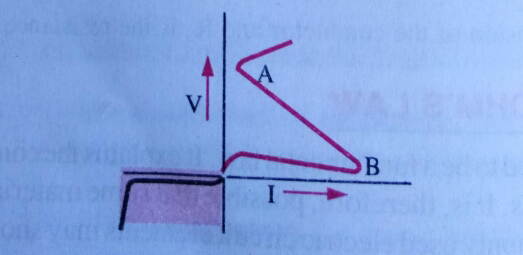Figure 1

(ii) Decrease of current is possible with increase in voltage :

The given figure shows the I-V curve for gallium arsenic. The curve BC shows that the current decreases when voltage increases. This fact cannot be explained with the help of ohms law .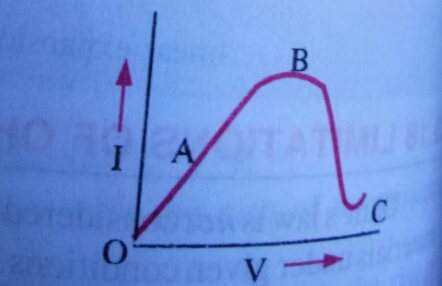Figure 2

Note:

Rectifier is a device which converts alternating current that is AC into direct current means DC.

(iii)  Passage of current Changes the nature of material that is non linear behavior between V and I :

This is an important point to understand. Resistance as we know is one type of property of a conductor which resists the flow of current through a conductor. It depends upon two factors like nature of material and temperature. If we increase the current flowing through a conductor , the conductor becomes hot and temperature doesn't remain same as  per the requirement of ohms law . So, in the situation like when the temperature of a conductor increases considerably, the conductors show non-linear behaviour which indicates that current I doesn't vary linearly with V . This situation is shown in the given figure. OA shows the actual graph between V and I which is a non-linear and OB is the desired graph between V and I as per ohms law for a given conductor.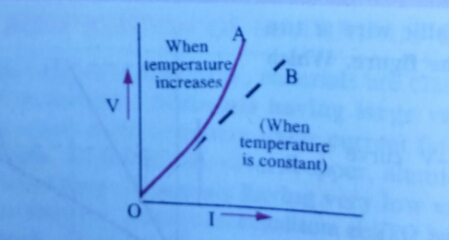Figure 3

## (iv) Current-Voltage relationship varies with the change of polarity of applied potential difference :

In a semiconductor diode , which can be used as a rectifier because it acts like a valve (unidirectional), current I depends on voltage V non-linearly. A diode offers low resistance to the flow of current in forward bias and high Resistance to the flow of current in reverse bias.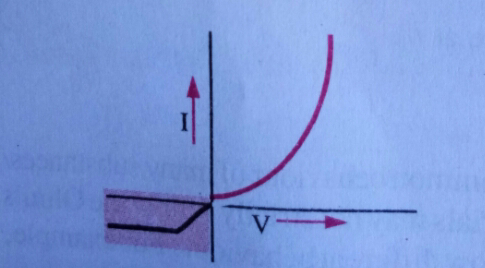Figure 4

If we look at the figure,  the shaded portion of the graph shows that inspite of increase of V in negative direction, rise of current is negligible. But if we talk about the unshaded portion, a small increase in positive V gives a high current. ohms law  clearly fails to explain it.

(v) Measurable current starts flowing only at a particular potential difference:

It is observed that in the water voltmeter that the graph between V and I is linear but current begins to flow only when V = V₀ . If we look at the figure, it shows that inspite of the presence of applied potential difference, current in the circuit is absent if V < V₀ . This observation is against ohms law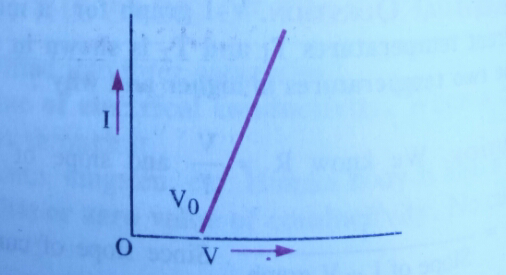Figure 5

#### OHMIC and NON - OHMIC circuit elements :-

The OHMIC Materials:-

Ohmic materials are those materials which obey ohms law . They are also known as ohmic circuit elements. For example, metals are ohmic resistors.

The graph between V and I that is voltage and current for ohmic materials is a straight line passing through the origin O such that I varies linearly with V.  Figure defines it best.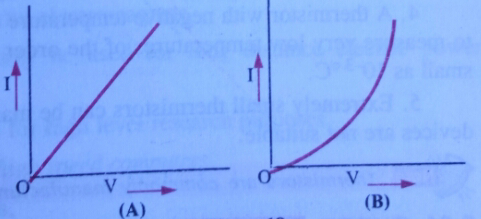#### OHMIC and NON - OHMIC elements

NON-OHMIC Materials :-

The materials or circuit elements which do not obey ohms law  are known as non-ohm or ohmic  materials or circuit elements.  For example,  vacuum tubes, semiconductor diodes, transistors, thermistors etc.  The graph shown in the figure between V and I for non-ohmic materials or circuit elements is not a straight line. In such cases, ratio of change of V to the change in I for a given voltage gives the dynamic Resistance of the circuit element.

i. e.                r = dv/dI

#### Conclusion:

In conclusion,  ohms law  is all about three topics named voltage, current and resistance that is V, I and R. It is an important concept of physics especially in the field of Current Electricity . The article has discussed all the information related to ohms law including the verification, derivation and limitations of the law .

1.This comment has been removed by the author.

2.This comment has been removed by the author.

3.Awesome post, I read an interesting topic here, and I like your style. Thanks a million, and please continue with effective work. Thank you for sharing this blog here. electrical test and tag

1.I will keep posting such informative articles.

4.I think this is one of the most important pieces of information for me. And I’m glad to read your article. Thank you for sharing. test and tag company

1.Thank you Sir

5.Thank you because you have been willing to share information with us. we will always appreciate all you have done here because I know you are very concerned with our. criminal defense law firm

1.You're always welcome 💞

6.I read the above article and I got some knowledge from your article. It's actually great and useful data for us. Thanks for share it.tag and test Melbourne

1.Thank you so much for your opinion.